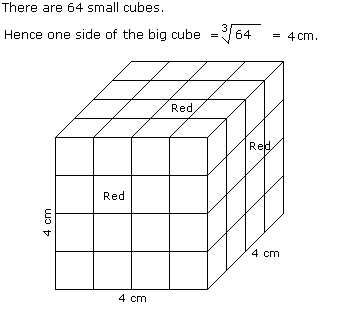# Verbal Reasoning - Cube and Cuboid

Exercise : Cube and Cuboid - Cube and Cuboid 4
Directions to Solve

The following questions are based on the information given below:

1. All the faces of cubes are painted with red colour.
2. The cubes is cut into 64 equal small cubes.

1.
How many small cubes have only one face coloured ?
4
8
16
24
Explanation:Number of small cubes having only one face coloured = (x - 2)2 x No. of faces

= (4 - 2)2 x 6

= 24

2.
How many small cubes have no faces coloured ?
24
8
16
0
Explanation:Number of small cubes having only one faces coloured = (x - 2)3

Here, x = side of big cube / side of small cube

x = 4 /1

x = 4

Required number = (4 -2)3

= 8

3.
How many small cubes are there whose three faces are coloured ?
4
8
16
24
Explanation:Number of small cubes having three faces coloured = No. of corners = 8

4.
How many small cubes are there whose two adjacent faces are coloured red ?
0
8
16
24